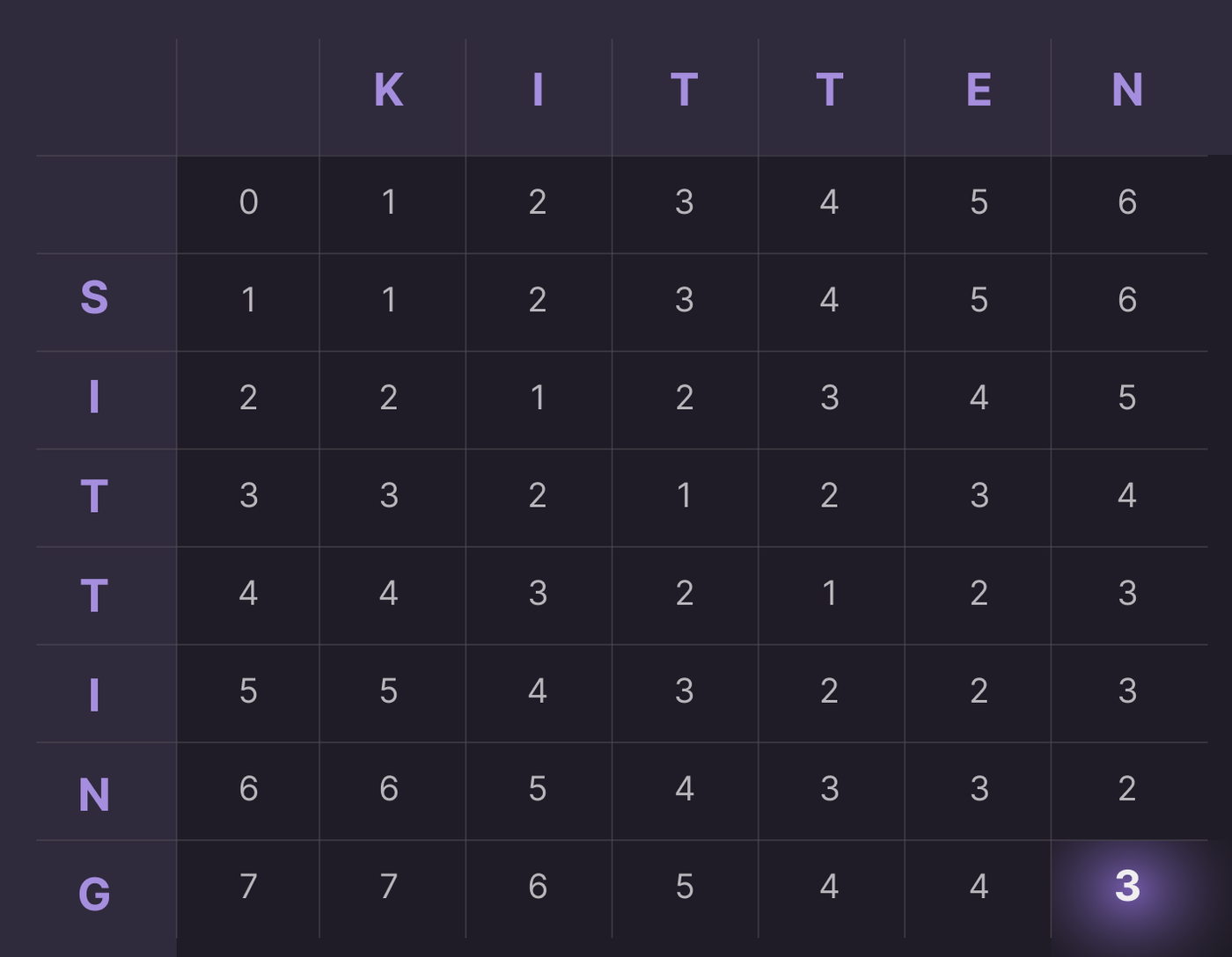Algorithms
April 01, 2023

# Typo no more. An in-depth guide to the Levenshtein edit distance

The Levenshtein edit distance, also known as the Levenshtein distance, is a measure of similarity between two strings, which quantifies the number of single-character edits required to change one string into the other. In this post, we will delve into the details of this algorithm, its applications, and its limitations. We will also explore various implementations and optimizations.

## Introduction

The Levenshtein edit distance was developed in 1965 by the Soviet mathematician Vladimir Levenshtein. The algorithm calculates the minimum number of single-character edits (insertions, deletions, and substitutions) needed to transform one string into another. This metric is widely used in various fields such as natural language processing, bioinformatics, data analysis, and search engines.

## Algorithm

There are several ways to compute the Levenshtein distance between two strings. We will discuss two popular methods: a naive recursive implementation and a dynamic programming-based implementation.

### Naive Recursive Implementation

The naive recursive implementation is based on the observation that the Levenshtein distance between two strings can be defined in terms of smaller substrings. Let’s denote the Levenshtein distance between strings `A` and `B` as `lev(A, B)`. The distance can be recursively defined as:

``````1function lev(A, B) {
2  if (A.length === 0) return B.length;
3  if (B.length === 0) return A.length;
4
5  const cost = A[A.length - 1] === B[B.length - 1] ? 0 : 1;
6
7  return Math.min(
8    lev(A.slice(0, -1), B) + 1,
9    lev(A, B.slice(0, -1)) + 1,
10    lev(A.slice(0, -1), B.slice(0, -1)) + cost
11  );
12}``````

This approach suffers from a high time complexity of `O(3^n)`, making it impractical for long strings.

### Dynamic Programming Implementation

The dynamic programming implementation of the Levenshtein distance algorithm, also known as the Wagner-Fisher algorithm, significantly reduces the time complexity by storing intermediate results in a matrix. This method has a time complexity of `O(mn)`, where `m` and `n` are the lengths of the input strings.

To compute the Levenshtein distance using dynamic programming, follow these steps:

1. Create a matrix `D` with dimensions `(m+1) x (n+1)`, where `m` and `n` are the lengths of the input strings `A` and `B`, respectively. Initialize the first row with values from `0` to `m` and the first column with values from `0` to `n`.
2. Iterate through the matrix from the second row and second column to the last row and last column. For each cell `(i, j)` in the matrix `D`, compute the cost `c`:
``1const c = A[i - 1] == B[j - 1] ? 0 : 1``

Update the value of the cell `(i, j)` with the minimum of the following:

``````1D[i - 1][j] + 1     // deletion
2D[i][j - 1] + 1     // insertion
3D[i - 1][j - 1] + c // substitution``````

The Levenshtein distance is the value in the bottom-right cell of the matrix `D`.

Here’s an example to illustrate the algorithm with input strings `A = "kitten"` and `B = "sitting"`:The Levenshtein distance between `kitten` and `sitting` is `3`, as shown in the bottom-right cell of the matrix.

And here’s a reference implementation of the dynamic programming algorithm written in JavaScript:

``````1function levenshteinDistance(A, B) {
2  const m = A.length;
3  const n = B.length;
4  const D = new Array(m + 1).fill(null).map(() => new Array(n + 1).fill(0));
5
6  for (let i = 0; i <= m; i++) D[i] = i;
7  for (let j = 0; j <= n; j++) D[j] = j;
8
9  for (let i = 1; i <= m; i++) {
10    for (let j = 1; j <= n; j++) {
11      const cost = A[i - 1] === B[j - 1] ? 0 : 1;
12
13      D[i][j] = Math.min(
14        D[i - 1][j] + 1,     // deletion
15        D[i][j - 1] + 1,     // insertion
16        D[i - 1][j - 1] + cost // substitution
17      );
18    }
19  }
20
21  return D[m][n];
22}``````

## Typo tolerance in Orama

Orama supports typo-tolerance thanks to an highly optimized Levenshtein implementation, and can be enabled using the following API:

``````1const results = await search(db, {
2  term: 'hllo wrld',
3  tolerance: 1,
4})``````

In that case, it will match all the terms where the edit distance between the term and the indexed words is equal to or less than `1`. When searching for `"hello, wrld"`, Orama will be able to understand the typo in `"wrld"`, and will retrieve the correct results including the word `"world"`.

## Conclusion

The Levenshtein edit distance is a powerful and versatile metric that quantifies the similarity between two strings by measuring the number of single-character edits needed to transform one string into the other. With applications in natural language processing, bioinformatics, data analysis, and search engines, this algorithm plays an important role in many domains.

Although the naive recursive implementation of the Levenshtein distance algorithm has a high time complexity, the dynamic programming-based approach greatly improves its efficiency, making it practical for a wide range of use cases.

Furthermore, the highly optimized Levenshtein implementation in Orama enables typo-tolerance in searches, enhancing the search experience for users.

In summary, the Levenshtein edit distance algorithm is a valuable tool for comparing and analyzing strings, enabling developers and researchers to build more accurate and effective solutions in various fields.

### Posted byMichele Riva

CTO @OramaSearch